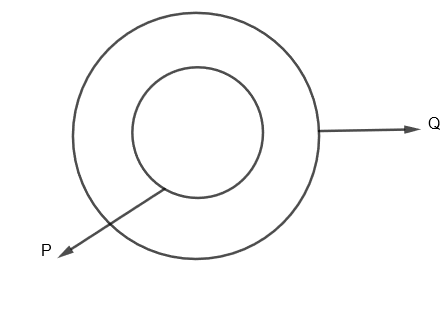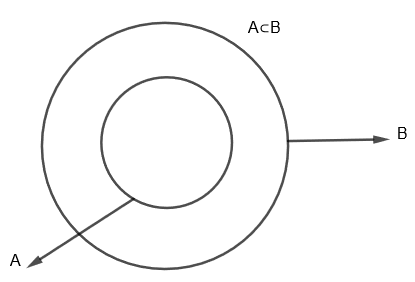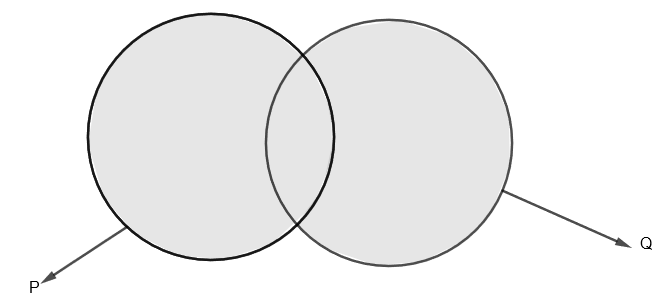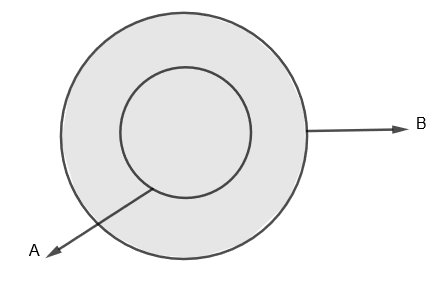Courses
Courses for Kids
Free study material
Free LIVE classes
More# If $A\subset B,n\left( A \right)=5$ and $n\left( B \right)=7$, then $n\left( A\cup B \right)=$_ _ _ _ _ _ _ _ _.(a) 5(b) 7(c) 2(d) 12

Last updated date: 25th Mar 2023
Total views: 308.4k
Views today: 7.86kVerified
308.4k+ views
Hint: Use the formula for union of two sets that is $n\left( A\cup B \right)=n\left( A \right)+n\left( B \right)-n\left( A\cap B \right)$. Now, since $A\subset B$, therefore put $n\left( A\cap B \right)=n\left( A \right)$, then use the given information that is $n\left( A \right)=5$ and $n\left( B \right)=7$.

Here, we are given two sets A and B such that $A\subset B$, $n\left( A \right)=5$ and $n\left( B \right)=7$. We have to find the value of $n\left( A\cup B \right)$.
Before proceeding with the question, we must know some of the terminologies related to sets.
First of all, a ‘set’ is a collection of well-defined and distinct objects. The most basic property of a set is that it has elements. The number of elements of a set, say A is shown by $n\left( A \right)$.
Here, in the question we have $n\left( A \right)=5$ and $n\left( B \right)=7$, that means the number of elements in set A is 5, while the number of elements in set B is 7.
Now, a ‘subset’ is a set which is contained in another set. We can also put it as, if we have a set P which is a subset of another set Q, then P is contained in Q as all the elements of set P are elements of Q. This relationship is shown by $P\subset Q$.
We can show it diagrammatically as,Here, $P\subset Q$, that means P is contained in Q as P is a subset of Q.
In question, we are given that $A\subset B$, that means that A is a subset of B or A is contained in B. We can show them asNow, union of two sets say P and Q is the set of elements which are in P, in Q or both P and Q. For example, if P = {1, 3, 5, 7} and Q = {1, 2, 4, 6, 7}, then union of P and Q which is shown as $P\cup Q=\left\{ 1,2,3,4,5,6,7 \right\}$
Diagrammatically, the shaded portion is $P\cup Q$ which is as followsThe formula for $n\left( P\cup Q \right)=n\left( P \right)+n\left( Q \right)-n\left( P\cap Q \right)$.
Here, $P\cap Q$ is the area common to both P and Q.
Now, in the given question, we have to find $n\left( A\cup B \right)$, that is, the number of elements in A union B.
We can show $A\cup B$ by a shaded portion which is as follows.Here, $A\subset B$ and $n\left( A \right)=5$ and $n\left( B \right)=7$.
Here, we can see that the portion common to the set A and B that is $\left( A\cap B \right)$ is nothing but set A. Therefore, here we have $n\left( A\cap B \right)=n\left( A \right)=5$.
As we know that $n\left( P\cup Q \right)=n\left( P \right)+n\left( Q \right)-n\left( P\cap Q \right)$, therefore to get $n\left( A\cup B \right)$, we will put A and B in place of P and Q respectively, we will get
$n\left( A\cup B \right)=n\left( A \right)+n\left( B \right)-n\left( A\cap B \right)$
Since, we have found that $n\left( A\cap B \right)=n\left( A \right)=5$.
Therefore we get, $n\left( A\cup B \right)=n\left( A \right)+n\left( B \right)-n\left( A \right)$
By putting the values of n (A) and n (B), we get,
$n\left( A\cup B \right)=5+7-5$
$n\left( A\cup B \right)=7$
Therefore, we get $n\left( A\cup B \right)=7$
Hence, option (b) is correct.

Note: Students must note that whenever $A\subset B$, that is A is subset of B, then $n\left( A\cup B \right)$, that is the number of elements in A union B is equal to number of elements in set B that is, $n\left( A\cup B \right)=n\left( B \right)$ when $A\subset B$. Also, some students make this mistake of writing $n\left( A\cup B \right)=n\left( A \right)+n\left( B \right)$ which is wrong. They must remember to subtract $n\left( A\cap B \right)$ as well. Hence, $n\left( A\cap B \right)=n\left( A \right)+n\left( B \right)-n\left( A\cap B \right)$.